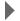Revision history[back]

# Shearing transform

Hi, In want to do a shearing transform of 2Q-1Q correlation spectra into a 1Q-1Q type projection. This is possible in NMRpipe using the macro shear.M, and it technically works fine.

## The macro is as follows:

shift = slope*(yLoc - offset);

p0 = 0.0; p1 = -360.0*shift;

(void) hilbert( rdata, idata, size );
(void) ift( rdata, idata, size );
(void) phase( rdata, idata, size, p0, p1 );
(void) fft( rdata, idata, size );

Now the "slope" and "offset" are the input. I think the slope value to be input should include somehow also the dwell time in the indirect dimension. However, i cannot figure out which units i should use for time...

Perhaps some of you have experience, could you please guide me therough the process...2 No.1 Revision posted Nov 23 '10 at 09:53Evgeny Fadeev 577●1

# Shearing transform

Hi, In want to do a shearing transform of 2Q-1Q correlation spectra into a 1Q-1Q type projection. This is possible in NMRpipe using the macro shear.M, and it technically works fine.

## The macro is as follows:

shift = slope*(yLoc - offset);

p0 = 0.0; p1 = -360.0*shift;

(void) hilbert( rdata, idata, size );
(void) ift( rdata, idata, size );
(void) phase( rdata, idata, size, p0, p1 );
(void) fft( rdata, idata, size );

Now the "slope" and "offset" are the input. I think the slope value to be input should include somehow also the dwell time in the indirect dimension. However, i cannot figure out which units i should use for time...

Perhaps some of you have experience, could you please guide me therough the process...3 No.2 Revision posted Jan 05 '11 at 10:18Evgeny Fadeev 577●1

# How to do a Shearing transformTransform in nmrPipe?

Hi, In want to do a shearing transform of 2Q-1Q correlation spectra into a 1Q-1Q type projection. This is possible in NMRpipe using the macro shear.M, and it technically works fine.

## The macro is as follows:

shift = slope*(yLoc - offset);

p0 = 0.0; p1 = -360.0*shift;

(void) hilbert( rdata, idata, size );
(void) ift( rdata, idata, size );
(void) phase( rdata, idata, size, p0, p1 );
(void) fft( rdata, idata, size );

Now the "slope" and "offset" are the input. I think the slope value to be input should include somehow also the dwell time in the indirect dimension. However, i cannot figure out which units i should use for time...

Perhaps some of you have experience, could you please guide me therough the process...